# 76. Dahlin controller¶

We will replicate the controller output in figure 17.11a. This notebook has a video commentary.

Note I am replicating these results using analytic methods to show that the artefacts are not numerical but rather fundamental to the calculations. If you simply want to simulate the action of a discrete controller on a continuous system, have a look at the Simple discrete controller simulation notebook.

:

import sympy
sympy.init_printing()
import tbcontrol
tbcontrol.expectversion('0.1.3')


# 77. Discretise the system¶

We need to find the corresponding z transform of the hold element and the system. Since $$H=1/s(1 - e^{-Ts})$$, we can find $$F=G/s$$, from there $$f(t)$$ and then work out the $$z$$ transform

:

s, t = sympy.symbols('s, t')
Gs = 1/(15*s**2 + 8*s + 1)
Gs

:

$$\frac{1}{15 s^{2} + 8 s + 1}$$
:

f = sympy.inverse_laplace_transform(Gs/s, s, t).simplify()
sympy.nsimplify(sympy.N(f)).simplify()

:

$$\theta\left(t\right) + \frac{3 e^{- \frac{t}{3}} \theta\left(t\right)}{2} - \frac{5 e^{- \frac{t}{5}} \theta\left(t\right)}{2}$$

We can see that $$f(t)$$ is the sum of 1 and two exponentials. It is easy to determine the corresponding z transforms from the table

Time domain Laplace-transform z-transform ($$b=e^{-aT}$$)
$$e^{-at}$$ $$\frac{1}{s+a}$$ $$\frac{1}{1-bz^{-1}}$$
:

z, q = sympy.symbols('z, q')


Now the sampling interval

:

T = 1 # Sampling interval

:

def expz(a):
b = sympy.exp(-a*T)
return 1/(1 - b*z**-1)

:

Fz = -5/2*expz(1/5)  + 3/2*expz(1/3) + 1/(1 - z**-1)

:

Fz

:

$$\frac{1.5}{1 - \frac{0.716531310573789}{z}} - \frac{2.5}{1 - \frac{0.818730753077982}{z}} + \frac{1}{1 - \frac{1}{z}}$$

Let’s see if we did that right

:

import tbcontrol.symbolic

:

def plotdiscrete(fz, N):
values = tbcontrol.symbolic.sampledvalues(fz, z, N)
times = [n*T for n in range(N)]
plt.stem(times, values)

:

import matplotlib.pyplot as plt
%matplotlib inline

:

import numpy

:

ts = numpy.linspace(0, 10, 300)

:

plotdiscrete(Fz, 10)
plt.plot(ts, tbcontrol.symbolic.evaluate_at_times(f, t, ts))

:

[<matplotlib.lines.Line2D at 0x1205b3ef0>]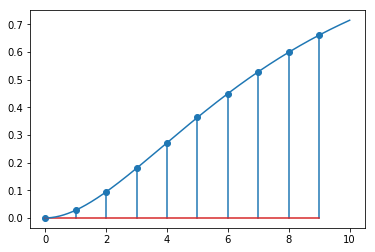Here is the transform of the system and the hold element. See the Discrete control notebook for the derivation.

:

HG_z = Fz*(1 - z**-1)


# 78. Dahlin Controller¶

The desired closed loop response is first order

:

θ = 0 # system dead time
λ = 1 # Dahlin's lambda
h = θ # Dahlin's h


First order response in eq 17-63

:

N = θ/T
A = sympy.exp(-T/λ)
yclz = (1 - A)*z**(-N-1)/(1 - A*z**-1)

:

K = (1/HG_z*yclz/(1 - yclz)).simplify()

:

K

:

$$\frac{1.0 \left(0.632120558828558 z^{2} - 0.970470713623842 z + 0.370831136111342\right)}{0.0279700831657292 z^{2} - 0.00455601047319787 z - 0.0234140726925314}$$
:

#K = 0.632/(1 - z*-1) * (1 - 1.5353*z**-1 + 0.5866*z**-2)/(0.028 + 0.0234*z**-1)


We will model the control response to a unit step in reference signal

:

rz = 1/(1 - z**-1) # unit step in z


Now we can calculate the z-domain version of the controller output

:

uz = K/(1 + K*HG_z)*rz

:

N = 10

:

plotdiscrete(uz, N)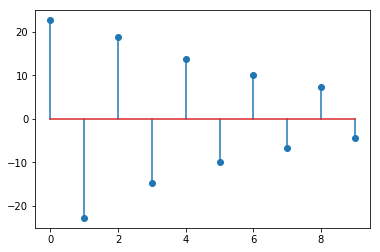This oscillating controller output is known as “ringing”. It is an undesirable effect.

# 79. Continuous response¶

By design the controlled variable follows an exponential at the sampling points.

:

yz = K*HG_z/(1 + K*HG_z)*rz

:

plotdiscrete(yz, N)
plt.plot(ts, 1 - numpy.exp(-ts/λ))

:

[<matplotlib.lines.Line2D at 0x120c51390>]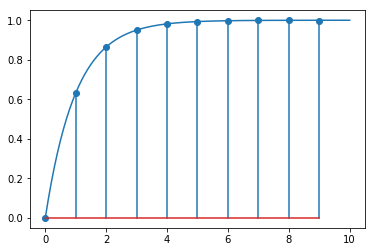But what does it really look like between data points? First, let’s construct the response of the system to a single sampling time length pulse input

:

p = f - f.subs(t, t-T)


Note that there is a slight issue with finding the value of this function at zero, so we avoid that point in plotting by starting at some small value which is not zero.

:

smallvalue = 0.001

:

sympy.plot(p, (t, smallvalue, 10))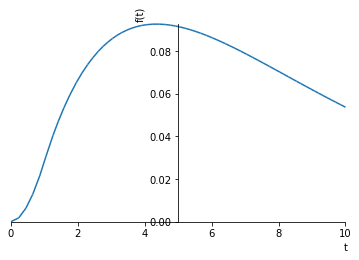:

<sympy.plotting.plot.Plot at 0x1209627f0>


Let’s get the values of the controller output as a list:

:

u = tbcontrol.symbolic.sampledvalues(uz, z, N)


Now, we calculate the output of the system as the sum of the various pulse inputs.

:

yt = numpy.zeros_like(ts)
for i in range(0, N):
yt += [float(sympy.N(u[i]*p.subs(t, ti-i + smallvalue))) for ti in ts]


Finally, we present the discrete system response, the designed response and the analytical continuous response on the same graph.

:

plotdiscrete(yz, 10)
plt.plot(ts, 1 - numpy.exp(-ts/λ))
plt.plot(ts, yt)

:

[<matplotlib.lines.Line2D at 0x12126f8d0>]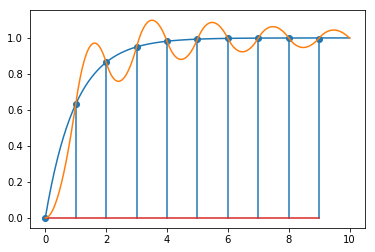[ ]: